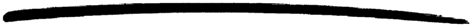# sets

sets

### MODULE SUMMARY

Functions for Set Manipulation

### DESCRIPTION

Sets are collections of elements with no duplicate elements. The representation of a set is not defined.

This module provides exactly the same interface as the module ordsets but with a defined representation. One difference is that while this module considers two elements as different if they do not match (=:=), ordsets considers two elements as different if and only if they do not compare equal (==).

### DATA TYPES

```set()
as returned by new/0

```

### EXPORTS

Types:

Set = set()

Returns a new empty set.

Types:

Set = term()

Returns true if Set is a set of elements, otherwise false.

Types:

Set = term()

Returns the number of elements in Set.

Types:

Set = set()
List = [term()]

Returns the elements of Set as a list.

Types:

List = [term()]
Set = set()

Returns an set of the elements in List.

Types:

Element = term()
Set = set()

Returns true if Element is an element of Set, otherwise false.

Types:

Element = term()
Set1 = Set2 = set()

Returns a new set formed from Set1 with Element inserted.

Types:

Element = term()
Set1 = Set2 = set()

Returns Set1, but with Element removed.

Types:

Set1 = Set2 = Set3 = set()

Returns the merged (union) set of Set1 and Set2.

Types:

SetList = [set()]
Set = set()

Returns the merged (union) set of the list of sets.

Types:

Set1 = Set2 = Set3 = set()

Returns the intersection of Set1 and Set2.

Types:

SetList = [set()]
Set = set()

Returns the intersection of the non-empty list of sets.

Types:

Set1 = Set2 = Set3 = set()

Returns only the elements of Set1 which are not also elements of Set2.

Types:

Set1 = Set2 = set()

Returns true when every element of Set1 is also a member of Set2, otherwise false.

Types:

Function = fun (E, AccIn) -> AccOut
Acc0 = Acc1 = AccIn = AccOut = term()
Set = set()

Fold Function over every element in Set returning the final value of the accumulator.

Types:

Pred = fun (E) -> bool()
Set1 = Set2 = set()

Filter elements in Set1 with boolean function Fun.

### See Also

stdlib 1.15
Copyright © 1991-2007 Ericsson AB